# Projective representations of symmetric and alternating groups

(diff) ← Older revision | Latest revision (diff) | Newer revision → (diff)

The classification of the projective representations of a finite group(cf. also Projective representation) was obtained by I. Schur [a9], [a10], who showed that over the complex fieldthe problem of determining all projective representations ofcan be reduced to determining the linear representations of stem extensions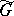of, called representation groups, by its Schur multiplier(cf. also Schur multiplicator). A standard reference is [a5].

In the case of the symmetric groups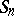and the alternating groups(cf. also Symmetric group; Alternating group), Schur [a11] further showed thatThe representation groups are not unique, for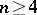there are two for; however, to determine the projective representations ofit suffices to consider one of these, which will be denoted by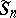; similarly,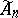is a representation group of. The non-linear representations ofand, that is, those representationsfor which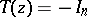,, whereis the generator of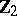are called spin representations. Schur [a10] classified the complex irreducible spin representations ofand,(and also the remaining non-linear projective representations for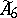and). Although more complicated, the classification of the spin representations follows the corresponding results for the linear representations of these groups. (cf. Representation of the symmetric groups). A standard reference is [a4], but see also [a12].

In this case, the irreducible spin representations are parametrized by the set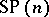of strict partitionsof, where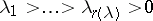. If(respectively,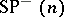) denotes the subset ofwhere the number of even parts is even (odd), then a complete list of irreducible spin representations is: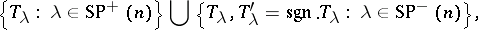whereis the sign representation of. The characters of these representations, called spin characters and denoted by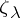and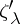, can take only non-zero values on the classes ofwhich are of cycle-type corresponding to partitions in, with all parts odd, and in. The values of the spin characters can be given explicitly in the case, but for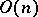can be determined from a class of symmetric functions introduced for this purpose by Schur and now called Schur-functions (cf. Schur-function) — these play an analogous role to that of Schur functions for linear representations of(cf. Schur functions in algebraic combinatorics). For each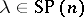, let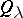denote the corresponding Schur-function; thenwhereis the value ofat the class of cycle-type,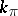is the order of that class and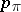is the corresponding power-sum symmetric function and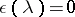oraccording as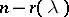is even or odd. If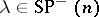, then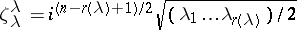andSchur also determined the dimension formula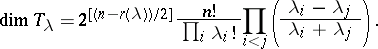The spin representations ofare now easily determined; if, then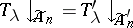is an irreducible spin representation and if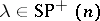, then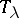splits into two conjugate irreducible spin representations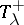and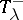of equal dimension and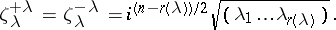All these results appeared in Schur's 1911 paper [a11] — the subject then lay dormant until the appearance of papers by A.O. Morris in the early 1960{}s [a6], [a7], where the combinatorial concepts of bars and bar lengths were introduced (cf. Schur-function); these correspond to the concepts of hooks and hook lengths in the linear case. Thus, the above dimension formula can be interpreted in terms of bar lengths:where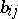denotes the bar length at theth node in the Young diagram corresponding to. Also, a recursion formula for calculating the irreducible spin characters analogous to the Murnaghan–Nakayama formula in the linear case was obtained in terms of these concepts. In all these formulas, as in the above dimension formula, the real difference is the complication added by the powers ofwhich appear.

Totally lacking until the 1990 work of M.L. Nazarov [a8] were explicit methods for constructing the irreducible spin matrix representations corresponding to each partition— these generalize the ones given by Schur for the partition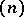. The method is comparable to the classical construction of the semi-normal form given by A. Young (cf. Representation of the symmetric groups). More recently, Nazarov has generalized Young's symmetrizer to the spin case. However, there are presently (2000) no analogues developed to Specht modules (cf. Specht module).

Some progress has been made on the modular spin representations of these groups. In 2001, the two papers [a2] and [a3] by J. Brundan and A. Kleshchev completely overturned the position. A conjecture corresponding to the classical Nakayama conjecture on the distribution of the spin characters into their-blocks has been proved — but, in general, the position here is even less understood than in the case of the modular ordinary representations.

See [a1] for the most recent developments.

How to Cite This Entry:
Projective representations of symmetric and alternating groups. Encyclopedia of Mathematics. URL: http://encyclopediaofmath.org/index.php?title=Projective_representations_of_symmetric_and_alternating_groups&oldid=14390
This article was adapted from an original article by A.O. Morris (originator), which appeared in Encyclopedia of Mathematics - ISBN 1402006098. See original article